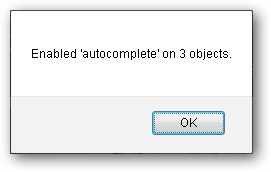# 如何强制您的浏览器记住密码### 为什么有些网站不允许我保存密码？### 修复：一个简单的JavaScript函数

JavaScript函数嵌入在下面的链接中。 您可以将链接拖动到书签栏或右键单击它，并将目标链接添加为书签。 一旦完成，只需单击书签将运行当前页面上的“允许密码保存”脚本。

 javascript：（function(){var％20ac，c，f，fa，fe，fea，x，y，z; ac =“autocomplete”; c = 0; f = document.forms; for（x = 0; xInternet Explorer中的用法### JavaScript源

```function() {
var ac, c, f, fa, fe, fea, x, y, z;
//ac = autocomplete constant (attribute to search for)
//c = count of the number of times the autocomplete constant was found
//f = all forms on the current page
//fa = attibutes in the current form
//fe = elements in the current form
//fea = attibutes in the current form element
//x,y,z = loop variables

ac = "autocomplete";
c = 0;
f = document.forms;

//cycle through each form
for(x = 0; x < f.length; x++) {
fa = f[x].attributes;
//cycle through each attribute in the form
for(y = 0; y < fa.length; y++) {
//check for autocomplete in the form attribute
if(fa[y].name.toLowerCase() == ac) {
fa[y].value = "on";
c++;
}
}

fe = f[x].elements;
//cycle through each element in the form
for(y = 0; y < fe.length; y++) {
fea = fe[y].attributes;
//cycle through each attribute in the element
for(z = 0; z < fea.length; z++) {
//check for autocomplete in the element attribute
if(fea[z].name.toLowerCase() == ac) {
fea[z].value = "on";
c++;
}
}
}
}

alert("Enabled " + ac + " on " + c + " objects.");
}```